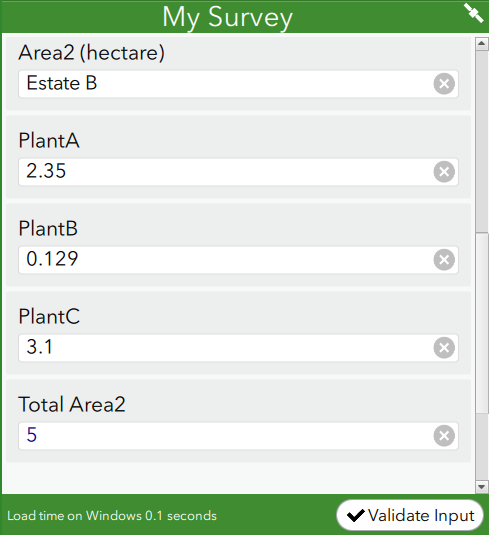English

# Problem: The number of output decimal places does not match the input decimal places in Survey123 Connect for ArcGIS

## Description

In Survey123 Connect for ArcGIS, most calculations return the output decimal places to match the input with the most decimal places. For example, the survey below is a calculation based on the formula: Plant1 + Plant2 + Plant3 = Total Area. The input data contains two, three, and one decimal places. The output returns a number with three decimal places, following the input with the most decimal places.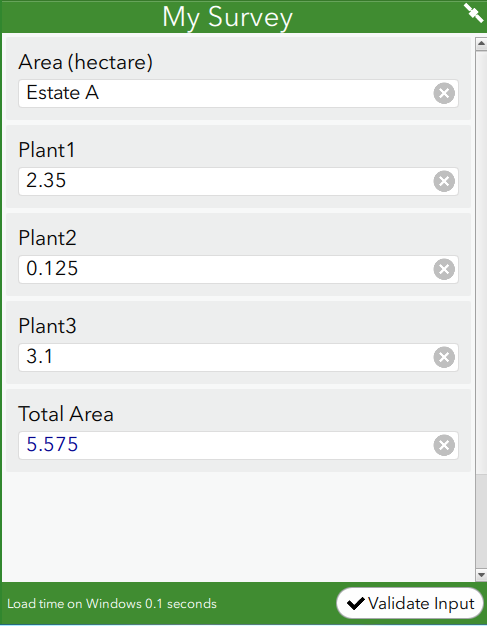In certain calculations, the output decimal places do not match the number of input decimal places. The image below shows input data with two, three, and one decimal places, but the output returns a number with 15 decimal places.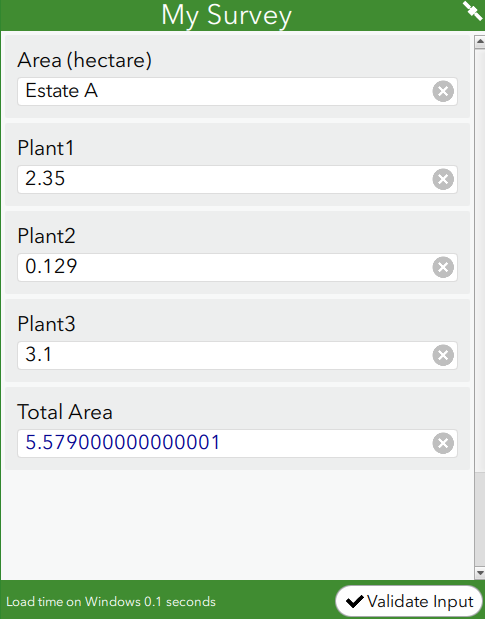## Cause

The calculation must be configured with the round function, to round the output according to the specified number of decimal places.

## Solution or Workaround

Follow the steps below to round to the desired number of output decimal places.

```Note:
These steps are for mathematical calculations involving the decimal data type.```
1. In the Survey123 Connect for ArcGIS XLS form, navigate to the calculation column, and enter the following formula:
`round(\${field_A} + \${field_B} + \${field_C}, decimal place)`

The image below shows the formula is configured in the XLS form using the round function, with the decimal places set to two.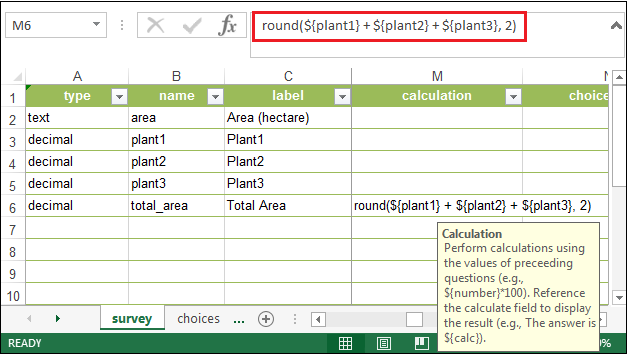2. Save the XLS form. This updates the Survey123 Connect for ArcGIS desktop.
3. In the Survey123 Connect for ArcGIS desktop, fill in the necessary fields. The image below shows the number of output decimal places matches the decimal places configured in the XLS form, which is two.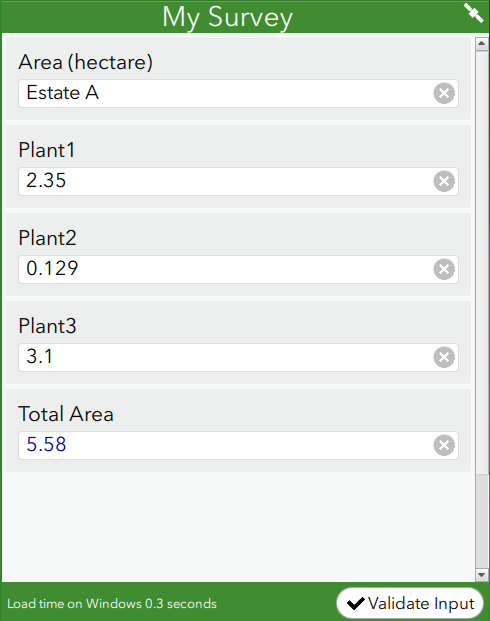As an alternative, follow the steps below to round the output to an integer, without any decimal places.
1. In the Survey123 Connect for ArcGIS XLS form, navigate to the calculation column, and enter the following formula:
`int(\${field_A} + \${field_B} + \${field_C})`

The image below shows the formula is configured in the XLS form using the int function.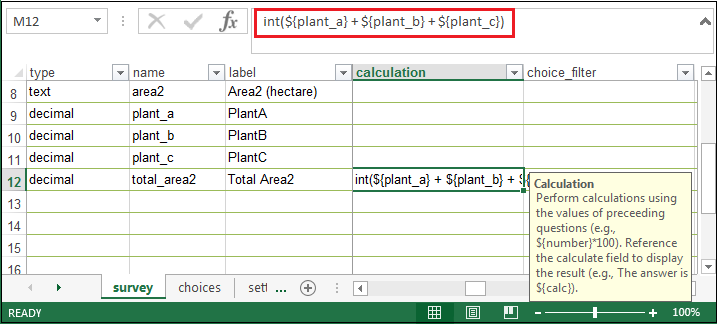2. Save the XLS form. This updates the Survey123 Connect for ArcGIS desktop.
3. In the Survey123 Connect for ArcGIS desktop, fill in the necessary fields. The image below shows the output is an integer, without any decimal places.